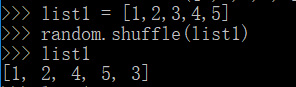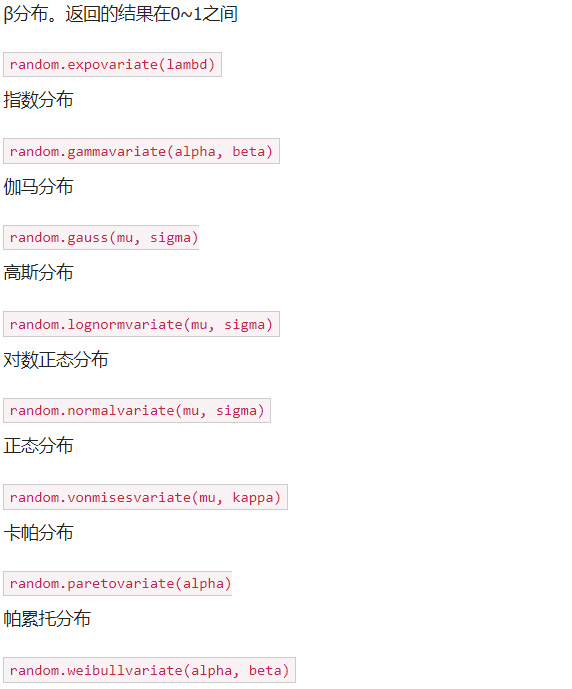﻿ python的random模块介绍[传智播客]

# python的random模块介绍[传智播客]

random模块介绍

1. 引入模块：import random

2. 使用模块中的某个功能：random.xxx(1, 10)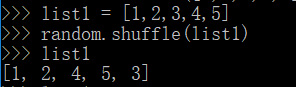random.random()方法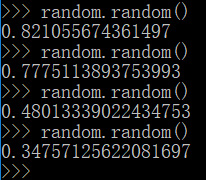random.randint()方法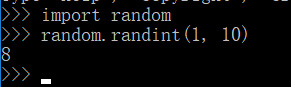random.randrange()方法

random.randrange(开始, 结束, 步长)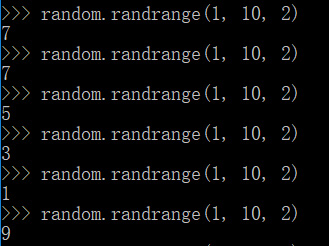random.uniform()方法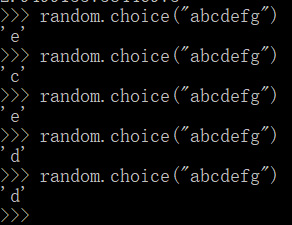random.choice()方法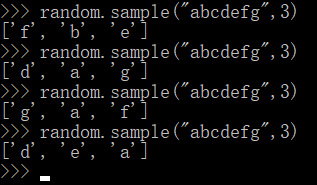random.sample()方法

random.shuffe()方法